## The Calendar Components

By maya On October 30th

1).- The Kin

The Maya year has a basic unit called Kin, a word that means day, Sun, etc. The Tzolkin calendar has a cycle of 20 day names combined with a cycle of 13 day numbers. Each of these 20 names has a glyph to represent it, these are: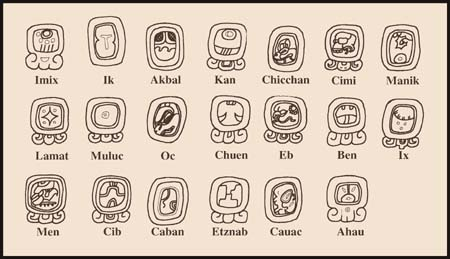2).- The Uinal

The Maya year is divided in 18 months, they are designated Uinal, each has a name and a corresponding glyph. Of these months, the first eighteen have twenty days and the last one, called Uayeb, has only five. The days within a month are numbered from 0 to 19 with the exception of Uayeb which is numbered from 0 to 4.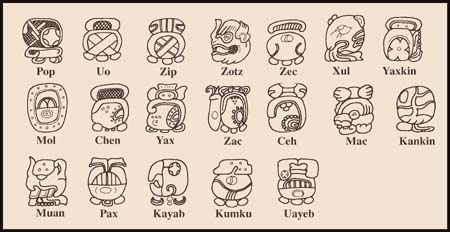3).-The Numbers

To write their dates the Maya used both the glyph corresponding to the different time periods and a number for each of them. The Maya developed a unique mathematical system that uses dots for units and bars for five units. The numbers can be written vertically or horizontally. They discovered and used the zero as well as a vigesimal positioning system, similar to the decimal positioning system used today. Its symbols and their Arabic equivalents are: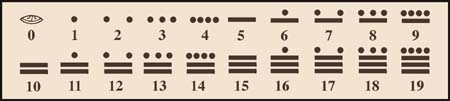Since the Maya numerical system is based on 20 units, when a number higher than 19 has to be written, a vertical positioning system that grows upwards is commonly used. Thus in order to write 20 they would place a zero in the bottom position with a dot on top of it. The dot in this place means one unit of the second order which is worth 20. To write 21, the zero would change to a dot and for the subsequent numbers the original 19 number count will follow in the first position. As they in turn reach 19 again, another units is added to the second position. This unit, for normal mathematical calculations, is worth 400 (20 x 20) , so to write 401 a dot goes in the first position , a zero in the second and a dot in the third. Positions higher than the third also grow multiplied by twenties from the previous ones. Only in the Maya calendric calculations is the third place unit worth 360 instead of 400, but after that, the rest of the positions also grow multiplied by twenties. Examples follow: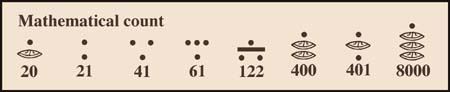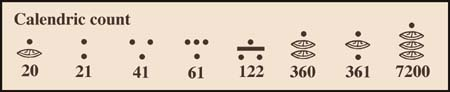As we mentioned previously the Maya set a fixed date to initiate their calendric calculations. This date is 4 Ahau 8 Kumku which in the Gregorian calendar used today corresponds to August 13, 3114 BC As we do today, to write any specific date they would consider the time elapsed since the beginning of their calendar. In order to do this the days were grouped into units like today’s years and centuries. Each of these units had a specific symbol (glyph). Their system is: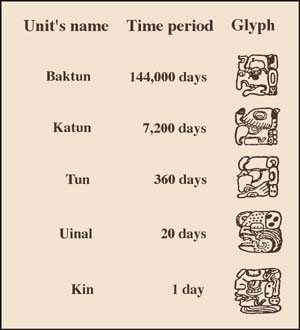Using these glyphs combined with numbers, any date can be written as the number of days that have passed since the beginning of the calendar. The Maya wrote their dates of importance in stone monuments called stelae some of which we can still see today. Some of them are like the stela with today’s date.

[Source: Maya Calendar]